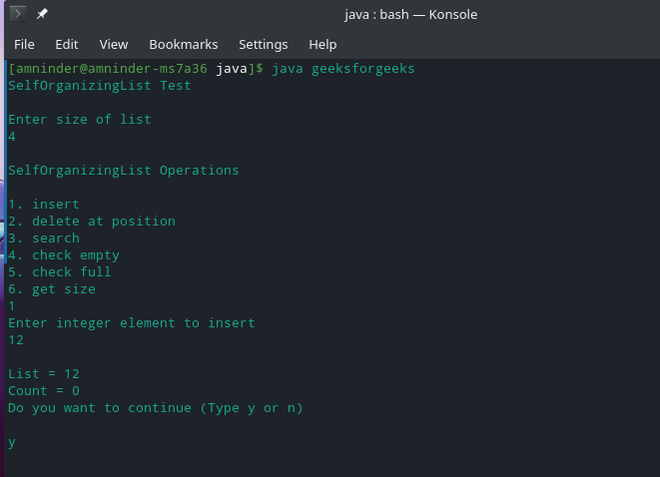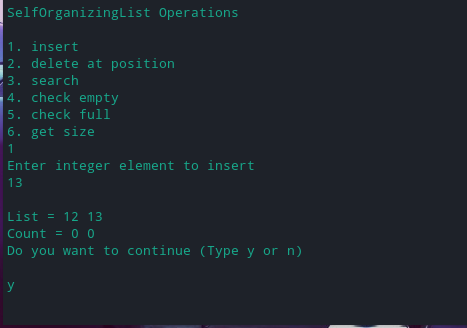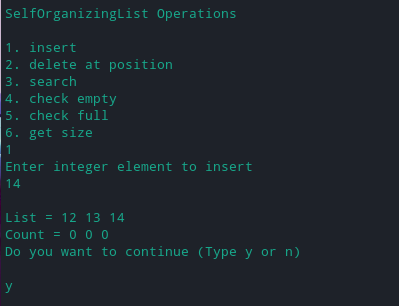# Implementing Self Organizing List in Java

• Difficulty Level : Medium
• Last Updated : 27 Oct, 2021

A Self-Organizing List is a list that modifies the order in which elements are stored based on the actual or expected access pattern. The goal is to achieve an ordering that keeps the most frequently sought elements closest to improve average access time. This property is also known as the locality of reference that brings the most frequently used items at the head of the list. This increases the probability of finding the item at the start of the list and those elements which are rarely used are pushed to the back of the list.

Techniques for Rearranging Nodes

While ordering the elements in the list, the access probabilities of the elements are not generally known in advance. This has led to the development of various heuristics to approximate optimal behavior. The basic heuristics used to reorder the elements in the list are,

1. Move to Front Method

This technique moves the element which is assessed to the head of the list.

At the t-th item selection:

if item i is selected:

move item i to head of the list

2. Count Method

In this technique, the number of times each node was searched for is counted i.e. every node keeps a separate counter variable which is incremented every time it is called.

init: count(i) = 0 for each item i

At t-th item selection:

if item i is searched:

count(i) = count(i) + 1

3. Transpose Method

This technique involves swapping an accessed node with its predecessor.

At the t-th item selection:

if item i is selected:

if i is not the head of list:

swap item i with item (i – 1)

## Java

 `// Java Program to Implement Self organizing List``import` `java.util.Scanner;` `class` `SelfOrganizingList {``    ``private` `int``[] list;``    ``private` `int``[] count;``    ``private` `int` `size;` `    ``// Constructor``    ``public` `SelfOrganizingList(``int` `listSize)``    ``{``        ``list = ``new` `int``[listSize];``        ``count = ``new` `int``[listSize];``        ``size = ``0``;``    ``}` `    ``// checks if list is empty``    ``public` `boolean` `isEmpty() { ``return` `size == ``0``; }` `    ``// checks if list is full``    ``public` `boolean` `isFull() { ``return` `size == list.length; }` `    ``// Makes list empty``    ``public` `void` `makeEmpty()``    ``{``        ``int` `l = list.length;``        ``list = ``new` `int``[l];``        ``count = ``new` `int``[l];``        ``size = ``0``;``    ``}` `    ``// returns the size of list``    ``public` `int` `getSize() { ``return` `size; }` `    ``// Function to insert element``    ``public` `void` `insert(``int` `val)``    ``{``        ``if` `(isFull()) {``            ``System.out.println(``"Error : List full!"``);``            ``return``;``        ``}``        ``list[size] = val;``        ``count[size] = ``0``;``        ``size++;``    ``}` `    ``// Function to remove element``    ``public` `void` `remove(``int` `pos)``    ``{``        ``pos--;``        ``if` `(pos < ``0` `|| pos >= size) {``            ``System.out.println(``"Invalid position "``);``            ``return``;``        ``}``        ``for` `(``int` `i = pos; i < size - ``1``; i++) {``            ``list[i] = list[i + ``1``];``            ``count[i] = count[i + ``1``];``        ``}``        ``size--;``    ``}` `    ``// Function to search for an element``    ``public` `boolean` `search(``int` `x)``    ``{``        ``boolean` `searchResult = ``false``;``        ``int` `pos = -``1``;``        ``for` `(``int` `i = ``0``; i < size; i++) {``            ``if` `(list[i] == x) {``                ``searchResult = ``true``;``                ``pos = i;``                ``break``;``            ``}``        ``}``        ``if` `(searchResult) {``            ``count[pos]++;``            ``int` `c = count[pos];``            ``for` `(``int` `i = ``0``; i < pos; i++) {``                ``if` `(count[pos] > count[i]) {``                    ``for` `(``int` `j = pos; j > i; j--) {``                        ``list[j] = list[j - ``1``];``                        ``count[j] = count[j - ``1``];``                    ``}``                    ``list[i] = x;``                    ``count[i] = c;``                    ``break``;``                ``}``            ``}``        ``}``        ``return` `searchResult;``    ``}` `    ``// prints list``    ``public` `void` `printList()``    ``{``        ``System.out.print(``"\nList = "``);``        ``for` `(``int` `i = ``0``; i < size; i++)``            ``System.out.print(list[i] + ``" "``);``        ``System.out.print(``"\nCount = "``);``        ``for` `(``int` `i = ``0``; i < size; i++)``            ``System.out.print(count[i] + ``" "``);``    ``}``}` `public` `class` `SelfOrganizingListTest {``    ``public` `static` `void` `main(String[] args)``    ``{``        ``Scanner scan = ``new` `Scanner(System.in);``        ``System.out.println(``"SelfOrganizingList Test\n"``);` `        ``// Creating object of class SelfOrganizingList``        ``System.out.println(``"Enter size of list "``);``        ``SelfOrganizingList list``            ``= ``new` `SelfOrganizingList(scan.nextInt());` `        ``char` `ch;` `        ``//  Perform list operations``        ``do` `{``            ``System.out.println(``                ``"\nSelfOrganizingList Operations\n"``);``            ``System.out.println(``"1. insert "``);``            ``System.out.println(``"2. delete at position"``);``            ``System.out.println(``"3. search"``);``            ``System.out.println(``"4. check empty"``);``            ``System.out.println(``"5. check full"``);``            ``System.out.println(``"6. get size"``);``            ``int` `choice = scan.nextInt();``            ``switch` `(choice) {``            ``case` `1``:``                ``System.out.println(``                    ``"Enter integer element to insert"``);``                ``list.insert(scan.nextInt());``                ``break``;``            ``case` `2``:``                ``System.out.println(``                    ``"Enter position to delete"``);``                ``list.remove(scan.nextInt());``                ``break``;``            ``case` `3``:``                ``System.out.println(``                    ``"Enter integer element to search"``);``                ``System.out.println(``                    ``"Search Result : "``                    ``+ list.search(scan.nextInt()));``                ``break``;``            ``case` `4``:``                ``System.out.println(``"Empty status = "``                                   ``+ list.isEmpty());``                ``break``;``            ``case` `5``:``                ``System.out.println(``"Full status = "``                                   ``+ list.isFull());``                ``break``;``            ``case` `6``:``                ``System.out.println(``                    ``"Size = "` `+ list.getSize() + ``" \n"``);``                ``break``;``            ``default``:``                ``System.out.println(``"Wrong Entry \n "``);``                ``break``;``            ``}` `            ``//  Display List``            ``list.printList();` `            ``System.out.println(``                ``"\nDo you want to continue (Type y or n) \n"``);``            ``ch = scan.next().charAt(``0``);``        ``} ``while` `(ch == ``'Y'` `|| ch == ``'y'``);``    ``}``}`

Output1.1 inserting element

Inserting element in the list :  121.2 inserting element

Inserting element in the list : 13Searching Element

Searching element  = 13

My Personal Notes arrow_drop_up